Disclosure: This post may contain affiliate links, meaning when you click the links and make a purchase, we receive a commission.

# Nominal Interest Rate vs. Effective Interest Rate Excel Formulas

There are different types of interest rates and it is prudent for every investor or potential investor to know the differences between interest rates. The nominal interest rate is the simplest of the interest rates. In the case of the nominal interest rate, it is the given interest rate or stated interest rate of a bond or loan. The nominal interest rate is also referred to as the coupon rate for fixed income investments.

In Excel, one can convert between the nominal annual interest rate and the effective annual interest rate using either the NOMINAL or the EFFECT Function.

The syntax of NOMINAL Function is: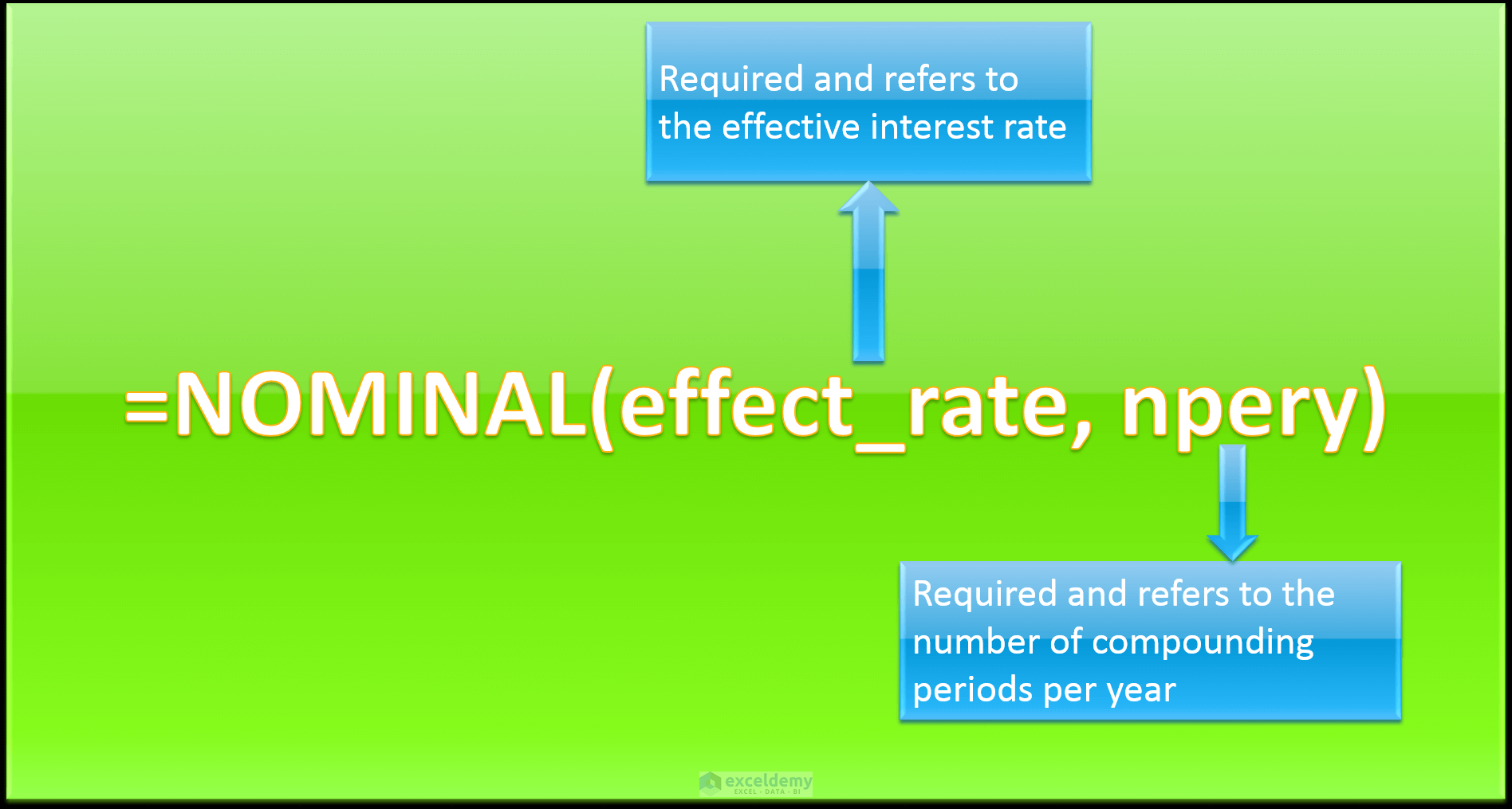The effective interest rate takes the effect of compounding into account. The difference between the nominal and effective rates widens as the compounding periods increase.

The effective annual interest rate is calculated using the EFFECT Function in Excel. The syntax for the EFFECT Function is: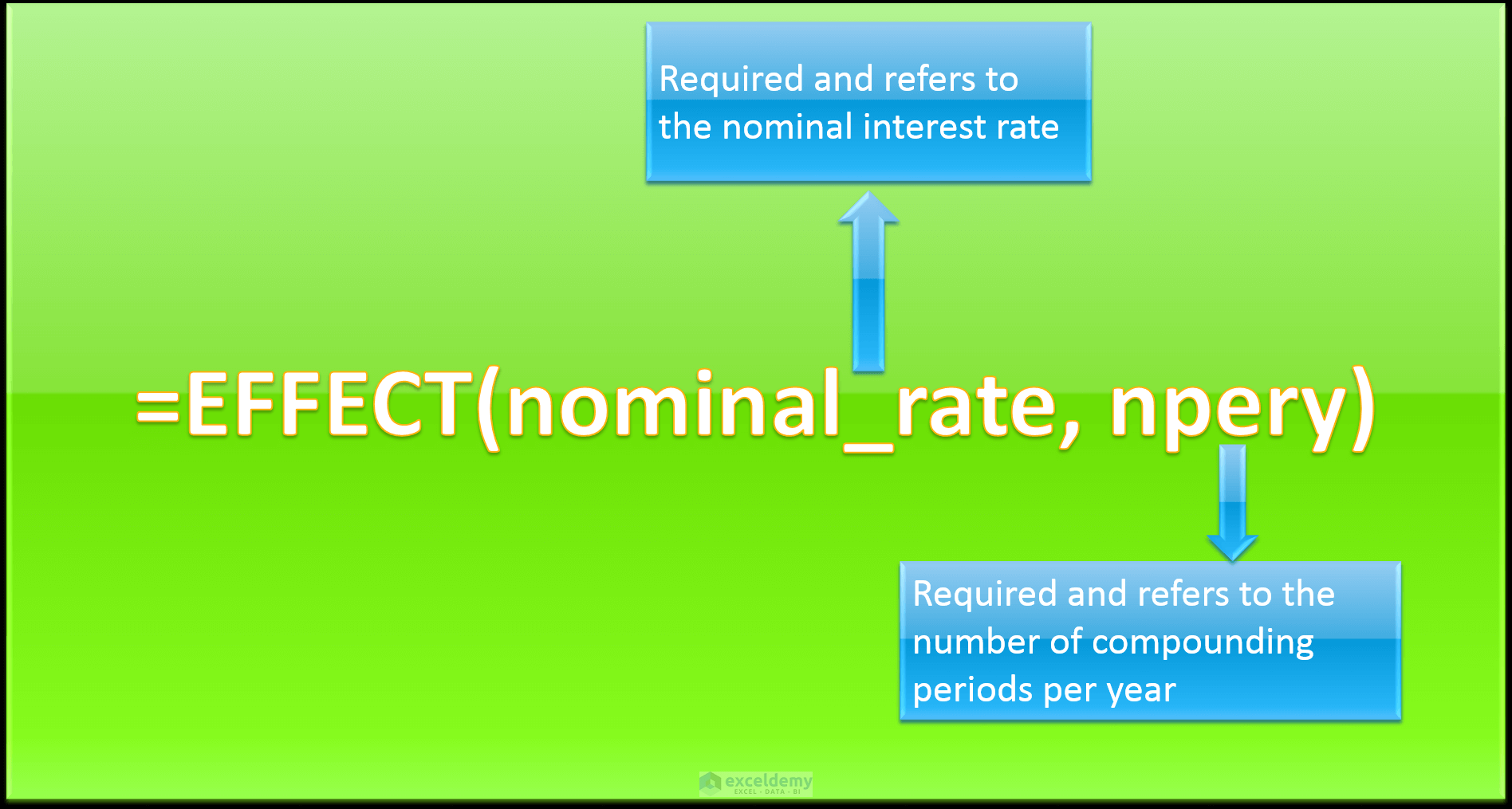## Calculating the nominal interest rate using the NOMINAL Function

The effective interest rate of a loan for a second-hand car is 2.5%, the number of compounding periods since its monthly payments required is 12. We want to figure out what the nominal annual interest rate is by utilizing the information given.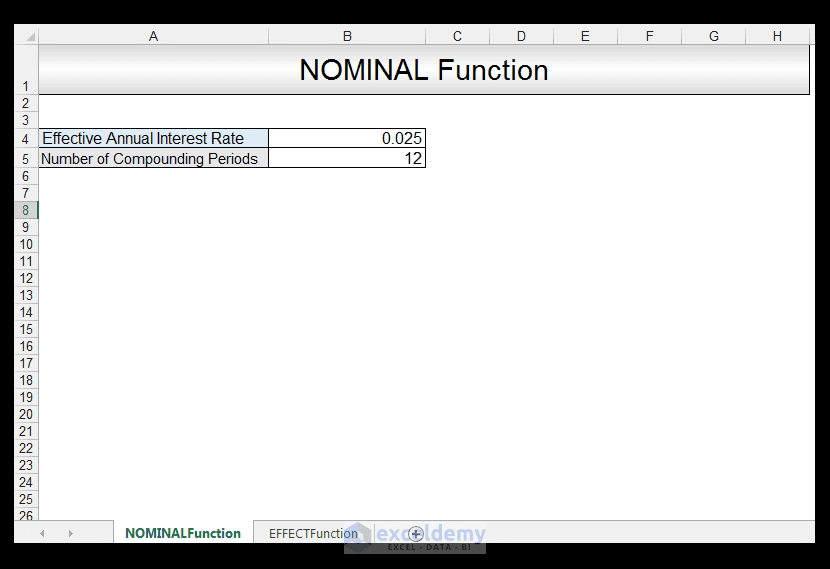1) In order to calculate the nominal annual interest rate, in Cell B6 we input the following formula:

=NOMINAL(B4, B5)2) Upon pressing CTRL-ENTER, a value of 0.0247 is returned, which is less than the effective interest rate, which is to be expected since the nominal interest rate does not include compounding.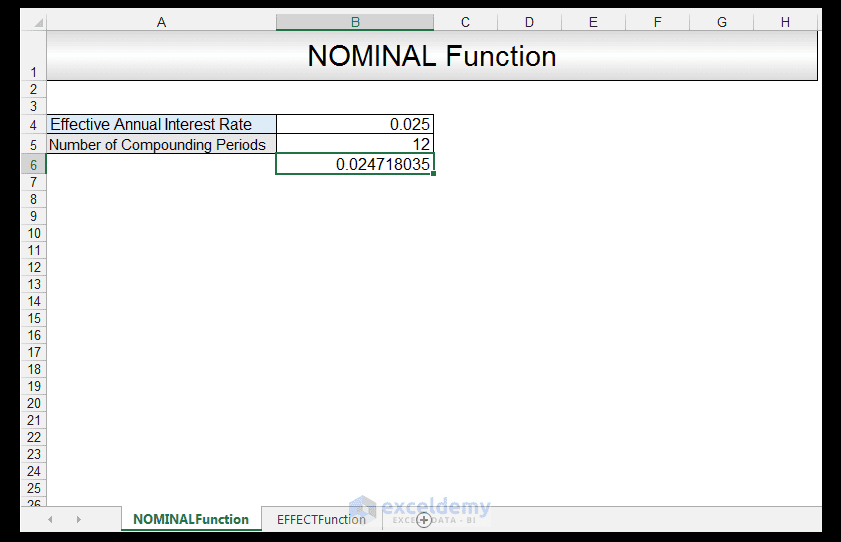## Calculating the effective annual interest rate using the EFFECT Function

The nominal annual interest rate of a loan is 7.8%, the number of compounding periods is 2. We want to figure out what the effective annual interest rate is, by utilizing the information given.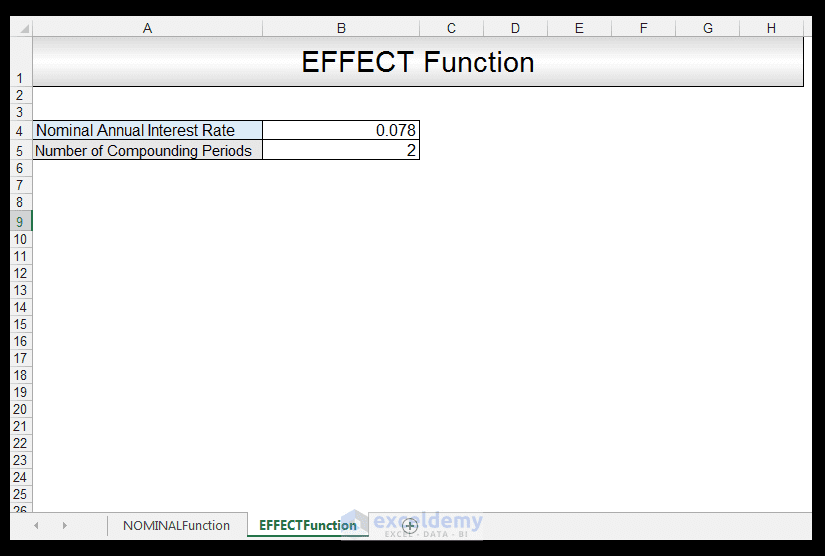1) In order to calculate the effective annual interest rate, in Cell B6 we input the following formula:

=EFFECT(B4, 2)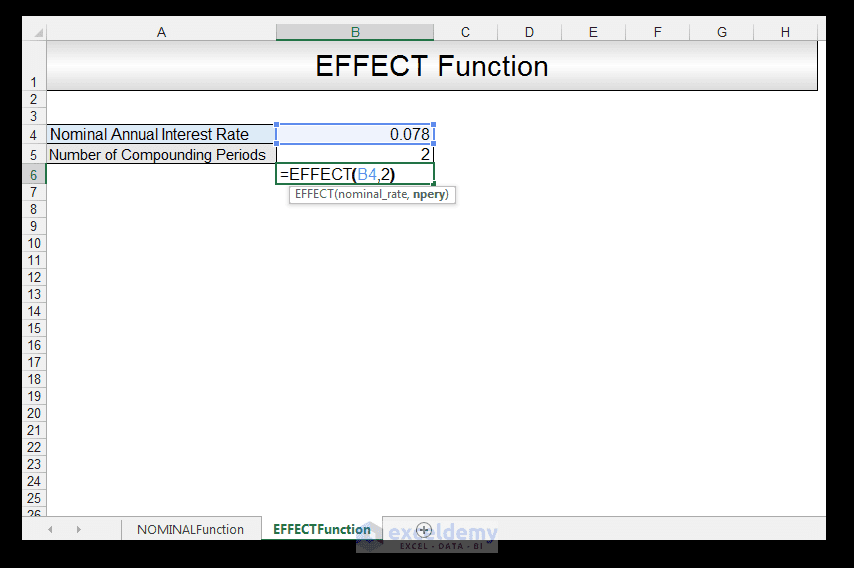2) Upon pressing CTRL-ENTER, a value of 0.0795 is returned, which is greater than the nominal rate since the effective annual interest rate includes compounding.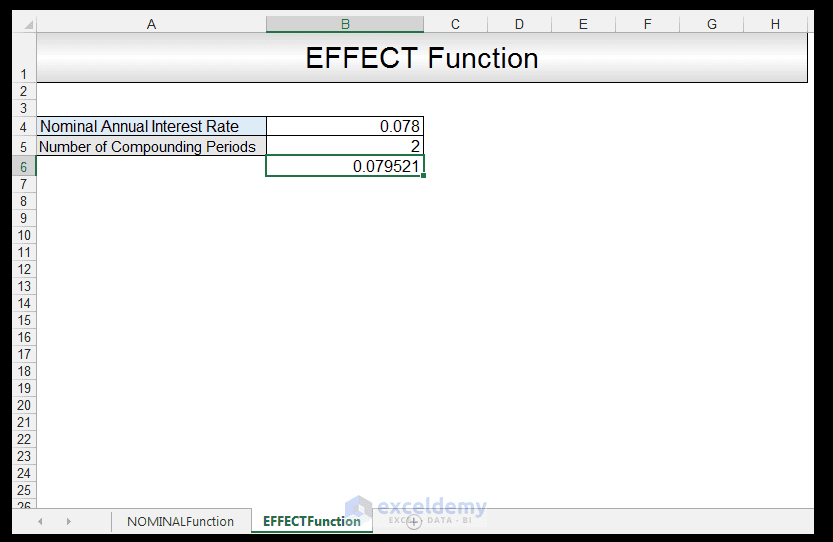## Conclusion

Some financial institutions often quote the nominal interest rate on loans since its fairly straightforward, however it can be slightly misleading since it doesn’t take into account the effect of compounding which the effective interest rate does. For small amounts and small time periods, the difference between the two is not heavily felt but for larger amounts and increasing time periods, the slightly larger effective interest rate is felt.

Please feel free to comment and tell us if you use nominal and effective interest rates in your financial spreadsheets.

Nominal-Versus-Effective-Interest-Rate